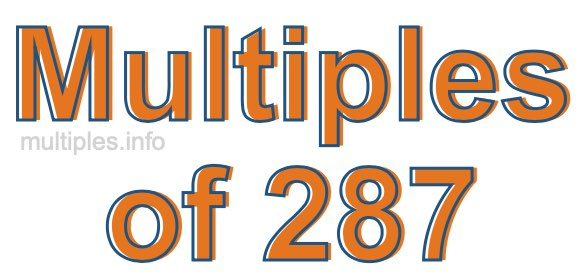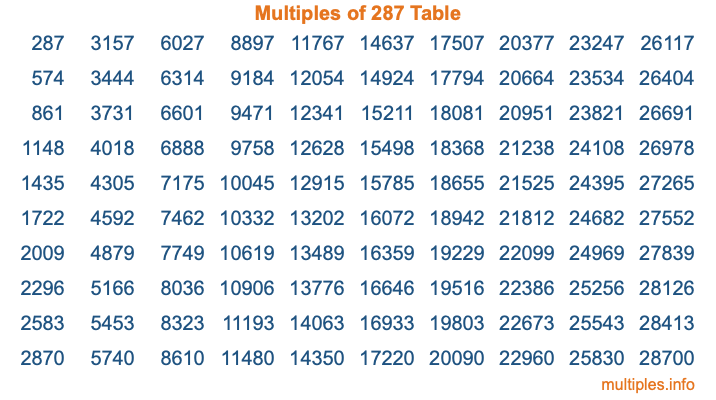Multiples of 287Welcome to the Multiples of 287 page. Here we will first teach you everything you will ever need to know about the multiples of 287, and then give you a study guide summary of everything we taught you to make sure you remember it all. Use this page to look up facts and learn information about the multiples of 287. This page will make you a multiples of two hundred eighty-seven expert!

Definition of Multiples of 287
Multiples of 287 are all the numbers that when divided by 287 equal an integer. Each of the multiples of 287 are called a multiple. A multiple of 287 is created by multiplying 287 by an integer.

Therefore, to create a list of multiples of 287, you start with 1 multiplied by 287, then 2 multiplied by 287, then 3 multiplied by 287, and so on for as long as you want. Thus, the list of the first five multiples of 287 is 287, 574, 861, 1148, and 1435. To see a larger list of multiples of 287, see the printable image of Multiples of 287 further down on this page. We also have a category where you can choose any nth multiple of 287.

Multiples of 287 Checker
The Multiples of 287 Checker below checks to see if any number of your choice is a multiple of 287. In other words, it checks to see if there is any number (integer) that when multiplied by 287 will equal your number. To do that, we divide your number by 287. If the the quotient is an integer, then your number is a multiple of 287.

Is  a multiple of 287?

Least Common Multiple of 287 and ...
A Least Common Multiple (LCM) is the lowest multiple that two or more numbers have in common. This is also called the smallest common multiple or lowest common multiple and is useful to know when you are adding our subtracting fractions. Enter one or more numbers below (287 is already entered) to find the LCM.

Check out our LCM Calculator if you need more details about the Least Common Multiple or if you need the LCM for different numbers for adding and subtraction fractions.

nth Multiple of 287
As we stated above, 287 is the first multiple of 287, 574 is the second multiple of 287, 861 is the third multiple of 287, and so on. Enter a number below to find the nth multiple of 287.

th multiple of 287

Multiples of 287 vs Factors of 287
287 is a multiple of 287 and a factor of 287, but that is where the similarities end. All postive multiples of 287 are 287 or greater than 287. All positive factors of 287 are 287 or less than 287.

Below is the beginning list of multiples of 287 and the factors of 287 so you can compare:

Multiples of 287: 287, 574, 861, 1148, 1435, etc.

Factors of 287: 1, 7, 41, 287

As you can see, the multiples of 287 are all the numbers that you can divide by 287 to get a whole number. The factors of 287, on the other hand, are all the whole numbers that you can multiply by another whole number to get 287.

It's also interesting to note that if a number (x) is a factor of 287, then 287 will also be a multiple of that number (x).

Multiples of 287 vs Divisors of 287
The divisors of 287 are all the integers that 287 can be divided by evenly. Below is a list of the divisors of 287.

Divisors of 287: 1, 7, 41, 287

The interesting thing to note here is that if you take any multiple of 287 and divide it by a divisor of 287, you will see that the quotient is an integer.

Multiples of 287 Table
Below is an image of the first 100 multiples of 287 in a table. The table is in chronological order, column by column. The first column has the first ten multiples of 287, the second column has the next ten multiples of 287, and so on.The Multiples of 287 Table is also referred to as the 287 Times Table or Times Table of 287. You are welcome to print out our table for your studies.

Negative Multiples of 287
Although not often discussed or needed in math, it is worth mentioning that you can make a list of negative multiples of 287 by multiplying 287 by -1, then by -2, then by -3, and so on, to get the following list of negative multiples of 287:

-287, -574, -861, -1148, -1435, etc.

Multiples of 287 Summary
Below is a summary of important Multiples of 287 facts that we have discussed on this page. To retain the knowledge on this page, we recommend that you read through the summary and explain to yourself or a study partner why they hold true.

There are an infinite number of multiples of 287.

A multiple of 287 divided by 287 will equal a whole number.

287 divided by a factor of 287 equals a divisor of 287.

The nth multiple of 287 is n times 287.

The largest factor of 287 is equal to the first positive multiple of 287.

287 is a multiple of every factor of 287.

287 is a multiple of 287.

A multiple of 287 divided by a divisor of 287 equals an integer.

287 divided by a divisor of 287 equals a factor of 287.

Any integer times 287 will equal a multiple of 287.

Multiples of a Number
Here you can get the multiples of another number, all with the same attention to detail as we did for multiples of 287 on this page.

Multiples of
Multiples of 288
Did you find our page about multiples of two hundred eighty-seven educational? Do you want more knowledge? Check out the multiples of the next number on our list!